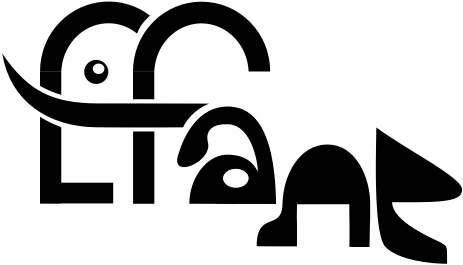# 2021/2022

• 2021-10-05
Algebraic values of the hypergeometric function

In this talk, I will study the general problem of when the value of the hypergeometric function $F(a,b;c;z)$ is algebraic, assuming $a$,$b$,$c$, and $z$ rational. The results involve modular forms and functions, complex multiplication, Shimura curves, and computer searches.